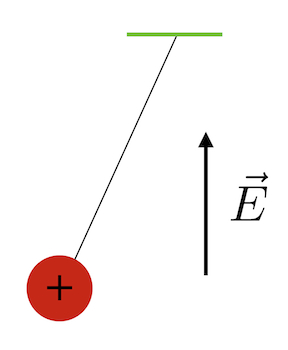# Charged pendulumLet the period of an uncharged pendulum be $t$. This pendulum is now charged with a charge of $+q$ and placed in a uniform electric field pointing vertically upward. If the new period of the pendulum is denoted by $T,$ which of the following is correct?

×